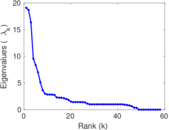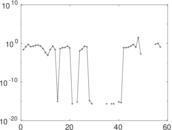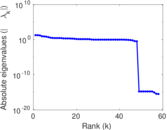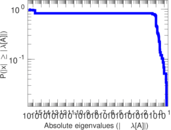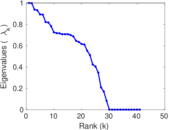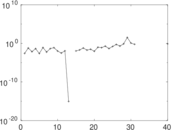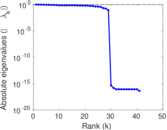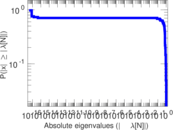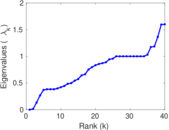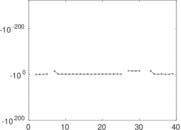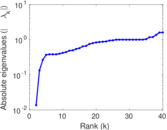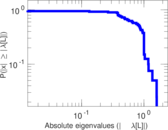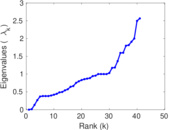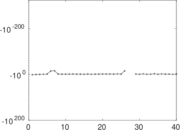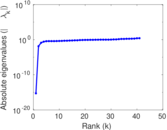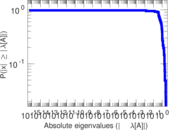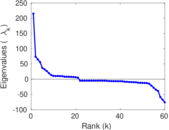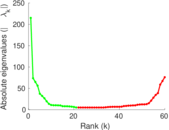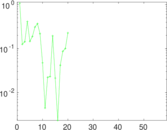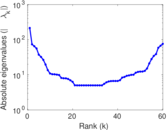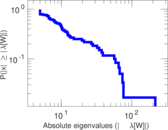# Wikipedia edits (kr)

This is the bipartite edit network of the Kanuri Wikipedia. It contains users and pages from the Kanuri Wikipedia, connected by edit events. Each edge represents an edit. The dataset includes the timestamp of each edit.

 Code `kr` Internal name `edit-krwiki` Name Wikipedia edits (kr) Data source http://dumps.wikimedia.org/ AvailabilityDataset is available for download Consistency checkDataset passed all tests Category Authorship network Dataset timestamp 2017-10-20 Node meaning User, article Edge meaning Edit Network formatBipartite, undirected Edge typeUnweighted, multiple edges Temporal dataEdges are annotated with timestamps

## Statistics

 Size n = 221 Left size n1 = 60 Right size n2 = 161 Volume m = 456 Unique edge count m̿ = 281 Wedge count s = 6,612 Claw count z = 160,316 Cross count x = 3,055,006 Square count q = 2,594 4-Tour count T4 = 47,890 Maximum degree dmax = 147 Maximum left degree d1max = 147 Maximum right degree d2max = 43 Average degree d = 4.126 70 Average left degree d1 = 7.600 00 Average right degree d2 = 2.832 30 Fill p = 0.029 089 0 Average edge multiplicity m̃ = 1.622 78 Size of LCC N = 149 Diameter δ = 10 50-Percentile effective diameter δ0.5 = 2.582 32 90-Percentile effective diameter δ0.9 = 6.359 17 Median distance δM = 3 Mean distance δm = 3.787 79 Gini coefficient G = 0.574 159 Balanced inequality ratio P = 0.288 377 Left balanced inequality ratio P1 = 0.197 368 Right balanced inequality ratio P2 = 0.368 421 Relative edge distribution entropy Her = 0.835 672 Power law exponent γ = 2.909 54 Tail power law exponent γt = 3.361 00 Tail power law exponent with p γ3 = 3.361 00 p-value p = 0.000 00 Left tail power law exponent with p γ3,1 = 1.931 00 Left p-value p1 = 0.040 000 0 Right tail power law exponent with p γ3,2 = 4.551 00 Right p-value p2 = 0.004 000 00 Degree assortativity ρ = −0.358 072 Degree assortativity p-value pρ = 6.337 69 × 10−10 Spectral norm α = 19.104 0 Algebraic connectivity a = 0.013 633 2 Spectral separation |λ1[A] / λ2[A]| = 1.023 28 Controllability C = 115 Relative controllability Cr = 0.545 024

## Plots

### Fruchterman–Reingold graph drawing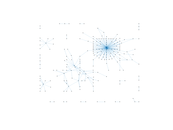### Degree distribution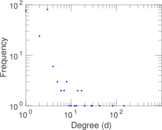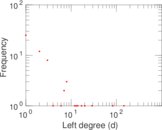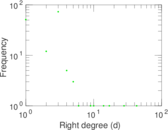### Cumulative degree distribution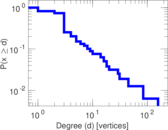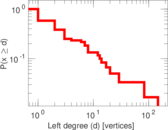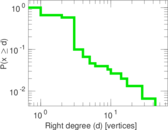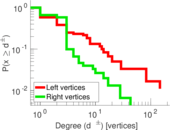### Lorenz curve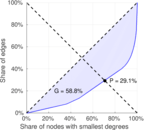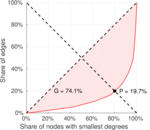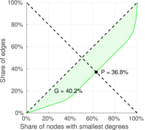### Spectral distribution of the adjacency matrix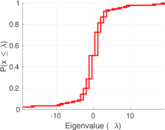### Spectral distribution of the normalized adjacency matrix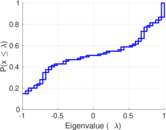### Spectral distribution of the Laplacian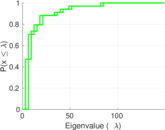### Spectral graph drawing based on the adjacency matrix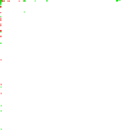### Spectral graph drawing based on the Laplacian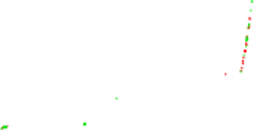### Spectral graph drawing based on the normalized adjacency matrix### Degree assortativity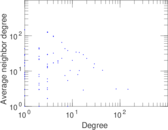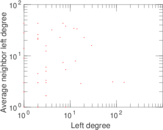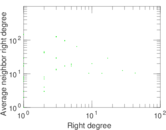### Zipf plot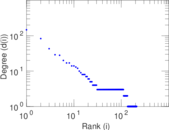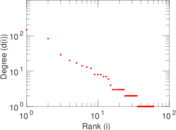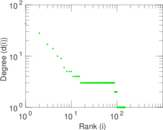### Hop distribution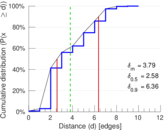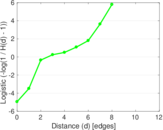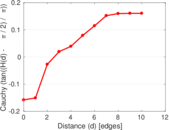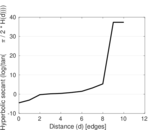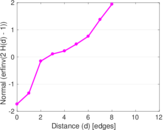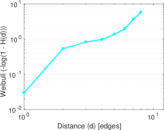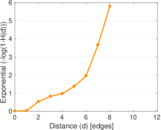### Double Laplacian graph drawing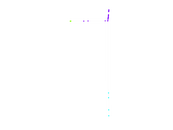### Delaunay graph drawing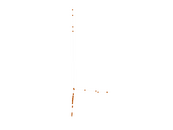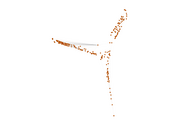### Edge weight/multiplicity distribution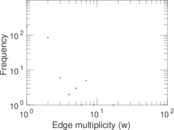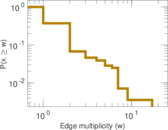### Temporal distribution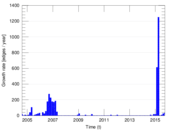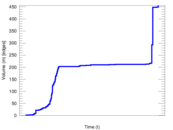### Temporal hop distribution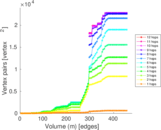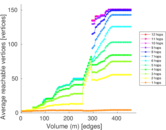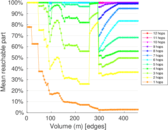### Diameter/density evolution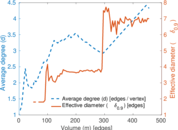### Matrix decompositions plots